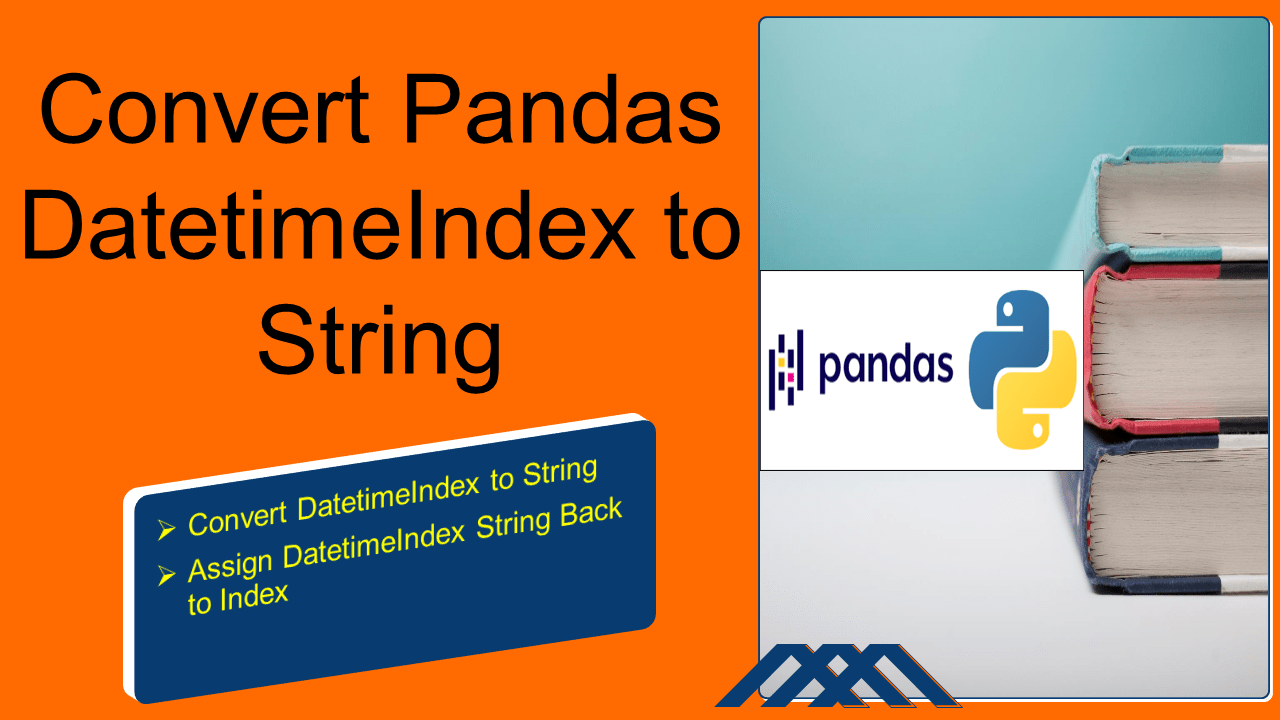# Convert Pandas DatetimeIndex to String

You can convert or cast pandas DatetimeIndex to String by using `pd.to_datetime()` and `DatetimeIndex.strftime()` functions. `DatetimeIndex` class is an Immutable ndarray which is used to store datetime64 data (internally stores as int64). When assigning a date field to an index it automatically converts into DatetimeIndex.

In this article, I will explain how to create an Index with DatatimeIndex and convert it to string format with multiple examples.

## 1. Quick Examples of DataTime Index to String

If you are in hurry, below are some quick examples of converting DatetimeIndex to String.

``````
# Below are some quick examples.

# Convert DatetimeIndex to String.
print(df.index.strftime('%m/%d/%Y, %r'))

# Example 2
print(pd.Series(df.index.format()))
``````

Now, Let’s create a pandas DataFrame with a few rows and columns, execute these examples and validate results. Here, `pandas.date_range()` function is used to create dates between two dates with frequency daily.

``````
# Create Pandas DataFrame.
import pandas as pd
df = pd.DataFrame({
'Courses':["Spark","PySpark","Spark"],
'Fee' :[22000,25000,23000],
'Duration':['30days','50days','35days']
})
df.index = pd.date_range('20210101','20210103',freq='D')
print(df)
print(type(df.index))
``````

Yields below output.

``````
Courses    Fee Duration
2021-01-01    Spark  22000   30days
2021-01-02  PySpark  25000   50days
2021-01-03    Spark  23000   35days
<class 'pandas.core.indexes.datetimes.DatetimeIndex'>
``````

## 2. Convert DatetimeIndex to String

As you see above, the type of `df.index` is DatetimeIndex, which you can use `DatetimeIndex.strftime()` to convert to a specific string format. The below example converts it into String with date format `'%m/%d/%Y, %r'`.

``````
# Convert DatetimeIndex to String.
print(df.index.strftime('%m/%d/%Y, %r'))
``````

Yields below output.

``````
Index(['01/01/2021, 12:00:00 AM', '01/02/2021, 12:00:00 AM',
'01/03/2021, 12:00:00 AM'],
dtype='object')
``````

Similarly, you can also use `df.index.format()` to convert DatetimeIndex.

``````
# Using Index.format
print(pd.Series(df.index.format()))
``````

Yields below output.

``````
0    2021-01-01
1    2021-01-02
2    2021-01-03
dtype: object
``````

## 3. Assign DatetimeIndex String Back to Index

You can assign this formatted DatetimeIndex String to Index but the index type would be Index with string values. Note that this is not a good practice as you would be losing the DatetimeIndex feature.

``````
df.index = df.index.strftime('%m/%d/%Y, %r')
print(df)
print(type(df.index))
``````

Yields output same as above.

``````
Courses    Fee Duration
01/01/2021, 12:00:00 AM    Spark  22000   30days
01/02/2021, 12:00:00 AM  PySpark  25000   50days
01/03/2021, 12:00:00 AM    Spark  23000   35days
<class 'pandas.core.indexes.base.Index'>
``````

## 4. Complete Examples of DataTimeIndex to String

``````
# Create Pandas DataFrame.
import pandas as pd
import numpy as np
technologies= {
'Courses':["Spark","PySpark","Spark"],
'Fee' :[22000,25000,23000],
'Duration':['30days','50days','35days']
}
df = pd.DataFrame(technologies)
df.index = pd.date_range('20210101','20210103',freq='D')
print(df)
print(type(df.index))

# Example 1
print(pd.Series(df.index.format()))

# Example 2
print(df.index.strftime('%m/%d/%Y, %r'))

# Example 3
df.index = df.index.strftime('%m/%d/%Y, %r')
print(df)
print(type(df.index))

# Example 4
# Create column with date
df['date'] = pd.date_range('20210101','20210103',freq='D')

# Column date would be of type datetime64[ns]
print(df)
print(df.date.dtypes)
``````

You can also find this example in the GitHub repository.

### Conclusion

In this article, I have explained how to convert DatetimeIndex to String format of pandas DataFrame index by using `pd.to_datetime()` and `DatetimeIndex.strftime()` functions with several examples.

### NNK

SparkByExamples.com is a Big Data and Spark examples community page, all examples are simple and easy to understand and well tested in our development environment Read more ..